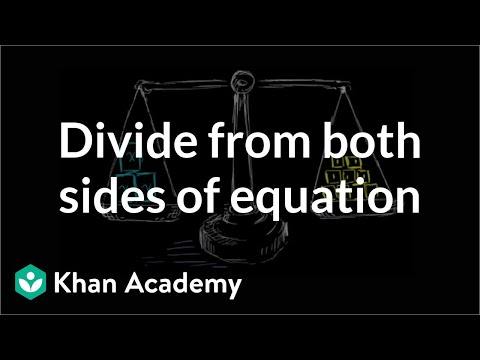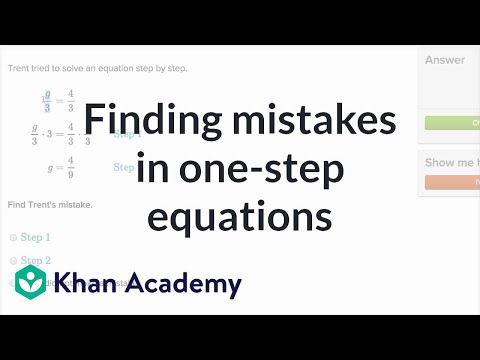# One-step multiplication equations (Full video)

Description: Remember that what you do to one side, you have to do to the other. Will you multiply or divide both sides to dump the fraction, x/a? Let's do it together. Created by Sal Khan and Monterey Institute for Technology and Education. So to solve for x, to figure out what the variable x must be equal to, we really just have to isolate it on the left-hand side of this equation. We have x divided by 3 is equal to 14. Obviously, x times 1/3 is going to be x/3.

### Other videos you might be interested in### Dividing both sides of an equation (Full video)